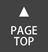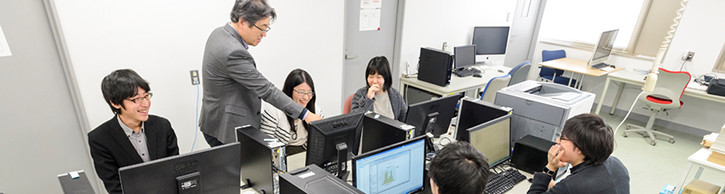# Department of Mathematical Informatics

## Summary

The Department of Mathematical Informatics conducts research and education on the foundation of informatics, with emphasis on the knowledge and skills of data analytics and mathematical modeling to analyze both natural and social phenomena. With the rapid development of information technology, such as the expansion of the Internet, the popularization of mobile devices, the improvement of measurement technologies as well as computer performance, society as a whole is being flooded with large amounts of information. Mathematical Informatics gives effective theories and methods for extracting and analyzing meaningful information, analyzing it and making decisions based on it.

In this major, we will build mathematical models to understand real world phenomena in nature and society, from the viewpoint of data analysis and informatics. By utilizing logical thinking and imagination to understand and develop mathematical models, which is fundamental to modeling, students will be able to develop a deeper understanding and interpretation of the phenomena through the use of informatics.
And through this, mathematical scientific methods are acquired for researchers and highly-skilled information engineers who play a core role in the application and development of informatics.

## Curriculum

Survey on Pure Mathematical Informatics 1・2
Survey on Applied Mathematical Informatics 1・2
Mathematical Logic 1・2
Discrete Mathematics 1・2
Quantum Information Theory 1・2
Number Theoretic Algorithms 1・2
Theory of Computability 1・2
Optimization 1・2
Computational Complexity 1・2
Foundations of Mathematics 1・2

## Units, Members

### Theoretical Informatics and Mathematics Unit

Students will analyze mathematical information structures in order to solve various problems inherent in natural, social, and unknown phenomena. Through this research, we aim to develop the foundational fields of informatics and to further the fundamental theory of informatics. In order to develop mathematical theory and to solve problems related to the basic characteristics of information and computers, we conduct research and education on mathematical methods such as mathematical logic, number theory and discrete mathematics.
Faculty Members
Prof.　YOSHINOBU, Yasuo　ONO, Hirotaka
Assoc. Prof.　SATOH, Junya　KIHARA, Takayuki

### Mathematical Modeling and Informatics Unit

Students will analyze mathematical information structures in order to solve various problems inherent in natural, social, and unknown phenomena. We conduct research and education on optimization theory, computational complexity theory, quantum information, etc. in order to elucidate unresolved problems in mathematical theory development and mathematical analysis of information generation, transmission and accumulation. Through this research, the aim is for students to construct mathematical informatics which can be applied to various fields related to information.
Faculty Members
Prof.　YAGIURA, Mutsunori　NISHIMURA, harumichi　BUSCEMI, Francesco
Assoc. Prof.　OTACHI, Yota
Assis. Prof.　KATO, Kohtaro　KURITA, Kazuhiro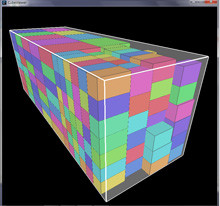A layout for the 3D box packing problem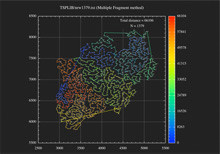A route for the traveling salesman problem with 1379
cities of state of Nordrhein-Westfalen (Germany)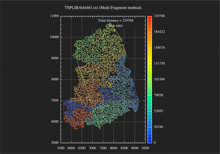A route for the traveling salesman problem with 4461 cities of five states of German